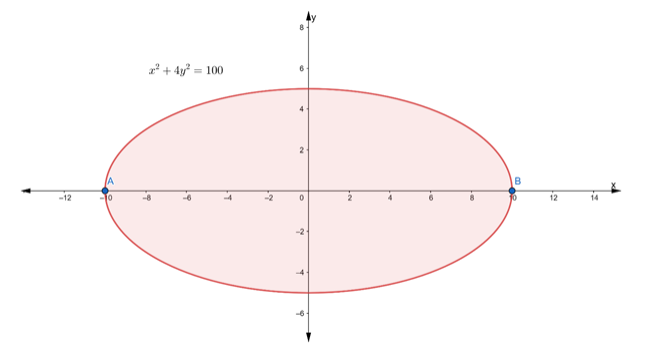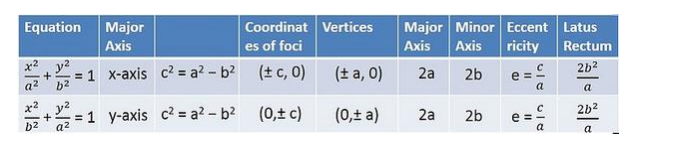# Find the value`
Question:

Find the

(i) lengths of major axes,

(ii) coordinates of the vertices,

(iii) coordinates of the foci,

(iv) eccentricity, and

(v) length of the latus rectum of each of the following ellipses.

$x^{2}+4 y^{2}=100$

Solution:Given:

$x^{2}+4 y^{2}=100$

Divide by 100 to both the sides, we get

$\frac{1}{100} x^{2}+\frac{4}{100} y^{2}=1$

$\frac{x^{2}}{100}+\frac{y^{2}}{25}=1$ …(i)Since, $100>25$

So, above equation is of the form,

$\frac{x^{2}}{a^{2}}+\frac{y^{2}}{b^{2}}=1$ …(ii)

Comparing eq. (i) and (ii), we get

$a^{2}=100$ and $b^{2}=25$

$\Rightarrow a=\sqrt{100}$ and $b=\sqrt{25}$

$\Rightarrow a=10$ and $b=5$

(i) To find: Length of major axes

Clearly, $a>b$, therefore the major axes of the ellipse is along $x$ axes.

$\therefore$ Length of major axes $=2 \mathrm{a}$

$=2 \times 10$

$=20$ units

(ii) To find: Coordinates of the Vertices

Clearly, a > b

$\therefore$ Coordinate of vertices $=(a, 0)$ and $(-a, 0)$

$=(10,0)$ and $(-10,0)$

(iii) To find: Coordinates of the foci

We know that,

Coordinates of foci $=(\pm c, 0)$ where $c^{2}=a^{2}-b^{2}$

So, firstly we find the value of $c$

$c^{2}=a^{2}-b^{2}$

$=100-25$

$c^{2}=75$

$c=\sqrt{75}$

$c=5 \sqrt{3} \ldots(l)$

$\therefore$ Coordinates of foci $=(\pm 5 \sqrt{3}, 0)$

(iv) To find: Eccentricity

We know that,

Eccentricity $=\frac{\mathrm{c}}{\mathrm{a}}$

$\Rightarrow \mathrm{e}=\frac{5 \sqrt{3}}{10}=\frac{\sqrt{3}}{2}[$ from $(\mathrm{I})]$

(v) To find: Length of the Latus Rectum

We know that,

Length of Latus Rectum $=\frac{2 b^{2}}{a}$

$=\frac{2 \times(4)^{2}}{5}$

$=\frac{32}{5}$